Courses
Courses for Kids
Free study material
Offline Centres
More

# Equal Chords and their Distances from CenterLast updated date: 27th Nov 2023
Total views: 58.2k
Views today: 1.58k## Equal Chords and their Distance from Center: Preface

Well, we see many round objects in daily life like coins, clocks, wheels, bangles, and many more. In this article, we will learn about the equal chords theorem i.e. the equal chords of a circle are equidistant from the center of the circle. And then will learn its converse too. After that, we discussed the theorem regarding the intersection of equal chords. At last, we will learn the diameter is the largest chord of the circle and we will solve examples to understand the concepts more easily.

## Perpendicular Bisector of a chord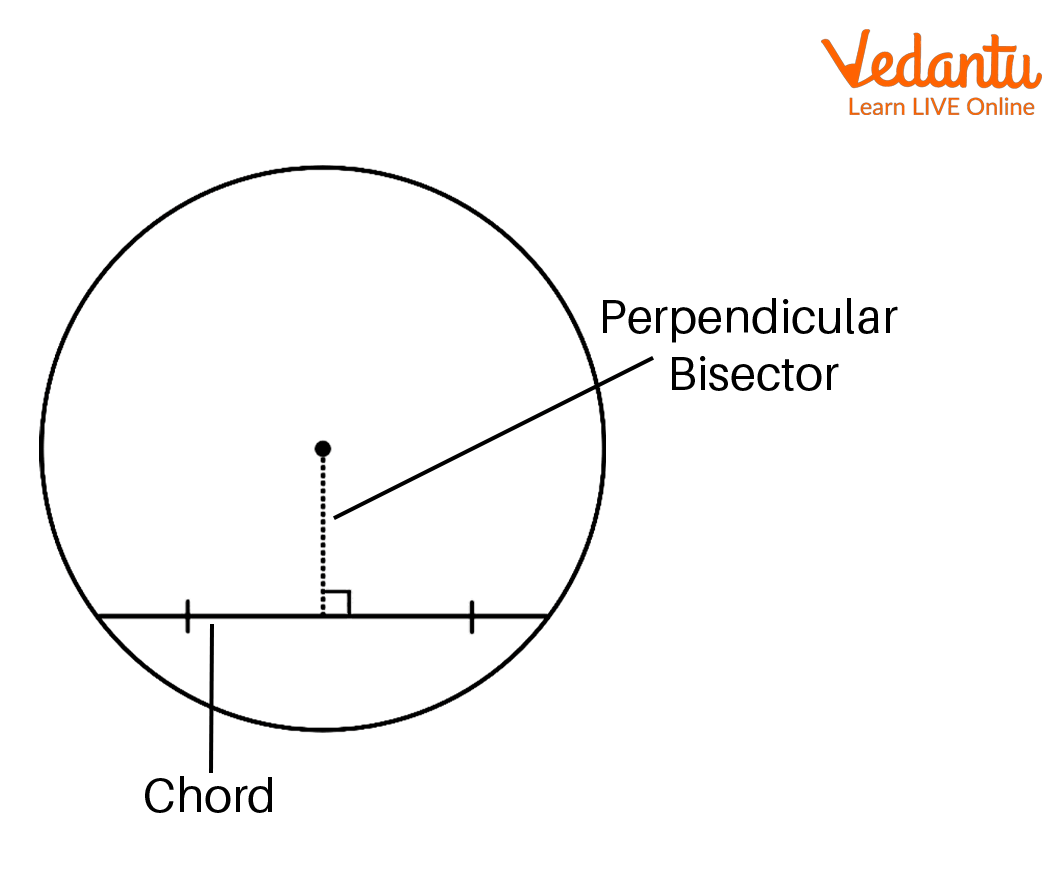Perpendicular Bisector of the Chord

Here, two points are joined to form a line segment which we call as Chord of the circle. The longest chord of a circle is called the diameter. When a line crosses the line at ${90^ \circ }$(perpendicular) and cuts in half (bisector) is called the perpendicular bisector of the chord. Also, this perpendicular bisector of the chord passes through the center of the circle. To represent a perpendicular line is ‘$\bot$’. Thus, the perpendicular drawn from the center of a circle to a chord bisects the chord.

## Chords and their Distance from the Center

As we know that there are infinite numbers of points on a line segment. We take a circle with center O having chord AB as shown below: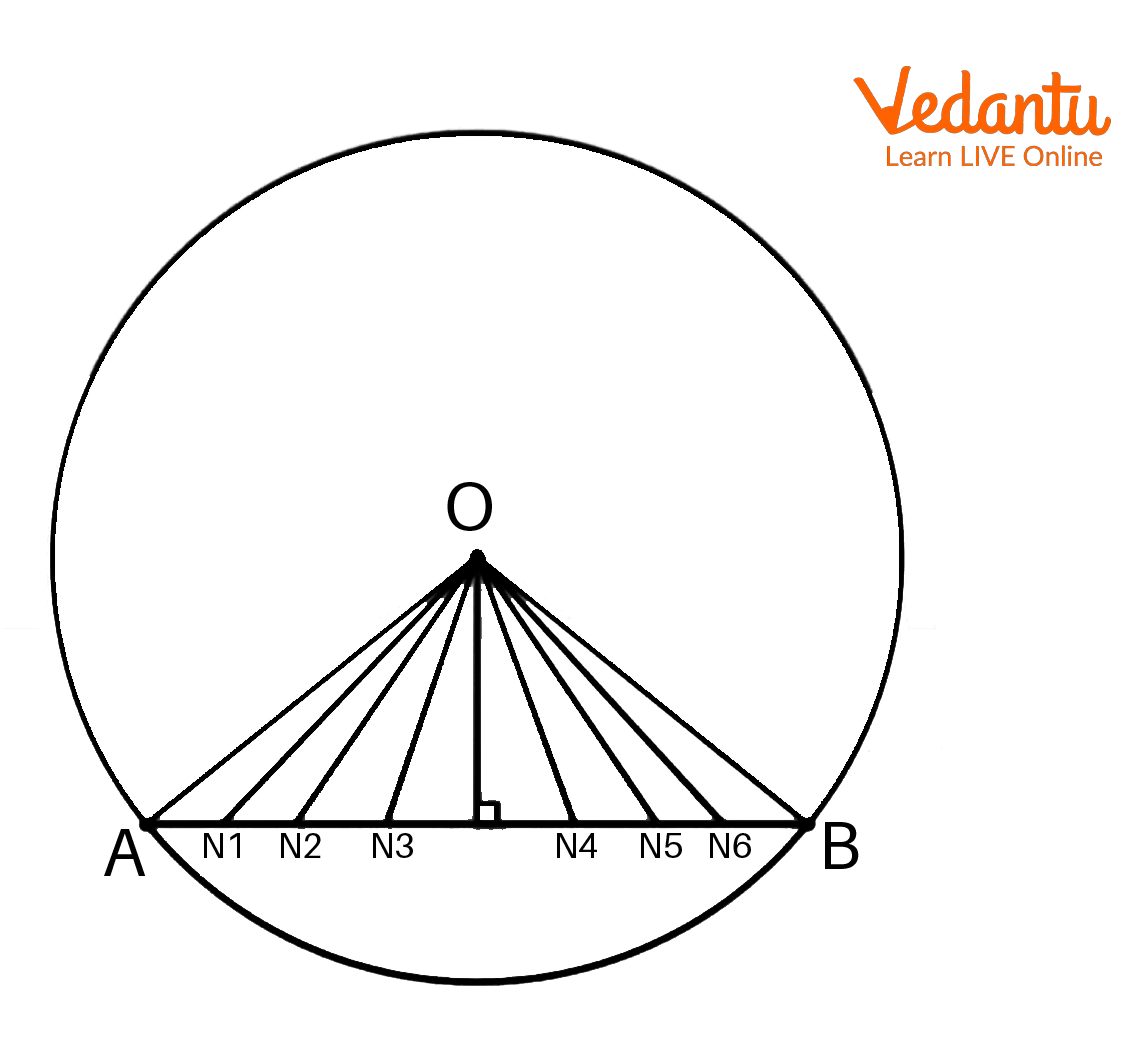Chord and their Distance from the Center

Next, to find the distance between the chord and the center O, if we join the infinite points on the line segment AB with O then we will get infinite line segments of different lengths. As we can see, line ON is perpendicular to AB and it has the shortest length i.e. $ON \bot AB$. This means the shortest length ON is the distance between O and AB. Thus we can say that the length of a perpendicular from a point to a line is the distance between the point from the line.

## Equal Chords and their Distance from the Center Theorem

Statement: Equal chords of a circle (or of congruent circles) are equidistant from the center (or centers).

Given: We have a circle with center O. AB and CD are chords that are equal i.e. AB = CD. Also, OX and OY are perpendiculars to AB and CD respectively

To prove: OX = OY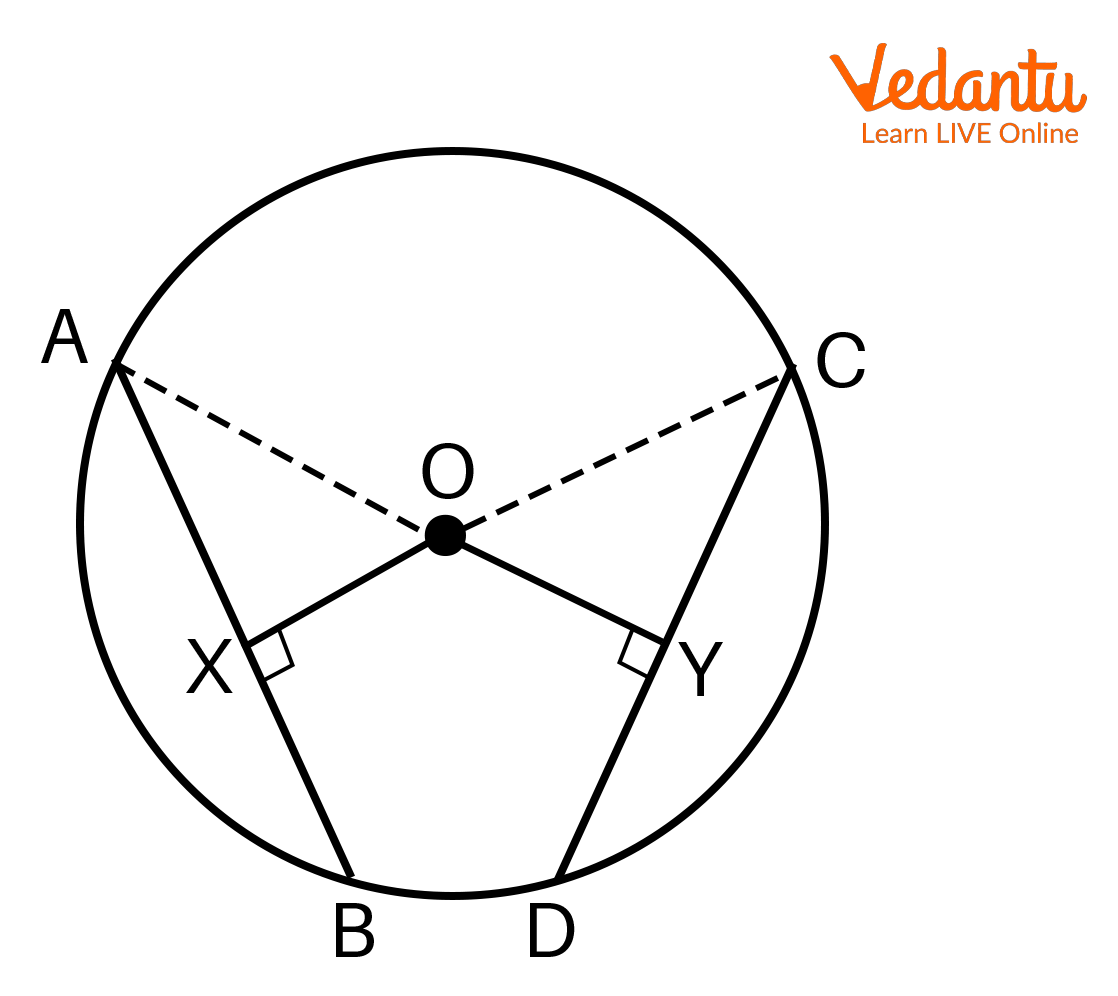Equal Chords and their Distance from the Center Theorem

Proof:

Draw OA and OC as shown in the figure.

As we know, perpendicular from the center to the chord bisects the chord.

Since, $OX \bot AB$

$\Rightarrow AX = BX = \frac{{AB}}{2}$ -------- (1)

Similarly for $OY \bot CD$

$\Rightarrow CY = DY = \frac{{CD}}{2}$ ---------- (2)

Next, given that AB = CD

$\Rightarrow \frac{{AB}}{2} = \frac{{CD}}{2}$

Using equations (1) and (2), we will get,

$\Rightarrow AX = CY$ -------- (3)

In $\Delta AOX$ and $\Delta COY$,

As both are right-angled triangles i.e. ${90^ \circ }$

$\Rightarrow \angle OXA = \angle OYC$

$\Rightarrow OA = OC$ (Both are the radii of the circle)

$\Rightarrow AX = CY$ (From (1))

So, RHS rule we can say that, $\Delta AOX \cong \Delta COY$

$\Rightarrow OX = OY$ (From CPCT rule)

Hence it is proved that equal chords of a circle are equidistant from the center.

## The Converse of this Above Theorem

Statement: Chords equidistant from the center of a circle are equal in length.

Given: We have a circle with center O. Also, AB and CD are chords of the circle where OX and OY are the distances from the center i.e. $OX \bot AB$ ($AX = BX = \frac{{AB}}{2}$) and $OY \bot CD$ ($CY = DY = \frac{{CD}}{2}$) respectively. And, OX = OY.

To prove: The chords are equal i.e. AB = CDConverse of Equal Chords and their Distance from the Center Theorem

Proof:

Draw OA and OC as shown in the figure.

In $\Delta AOX$ and $\Delta COY$

As both are right-angled triangles i.e. ${90^ \circ }$

$\Rightarrow \angle OXA = \angle OYC$

$\Rightarrow OA = OC$ (Both are the radii of the circle)

$\Rightarrow OX = OY$ (Given)

So, from RHS rule we can say that, $\Delta AOX \cong \Delta COY$

$\Rightarrow AX = CY$ (From CPCT rule) ------- (1)

Next, perpendiculars from the center to the chord bisect the chord of the circle.

So, for the chord AB:

$\therefore$ $OX \bot AB$

$\Rightarrow$ X bisects AB

$\Rightarrow AX = BX = \frac{{AB}}{2}$ ------ (2)

And, for the chord CD:

$\therefore$$OY \bot CD$

$\Rightarrow$ Y bisects CD

$\Rightarrow CY = DY = \frac{{CD}}{2}$ ------ (3)

Lastly, from (1), we have:

$\therefore AX = CY$

$\Rightarrow \frac{{AB}}{2} = \frac{{CD}}{2}$ (From (2) and (3))

$\Rightarrow AB = CD$

Hence it is proven.

## The Intersection of Equal Chords

Statement: If two equal chords of a circle intersect within the circle. Prove that, the segments of one chord are equal to the corresponding segments of another chord.

Given: AB = CD and both the chords intersect at point E in the circle.

To prove: AE = ED and CE = BE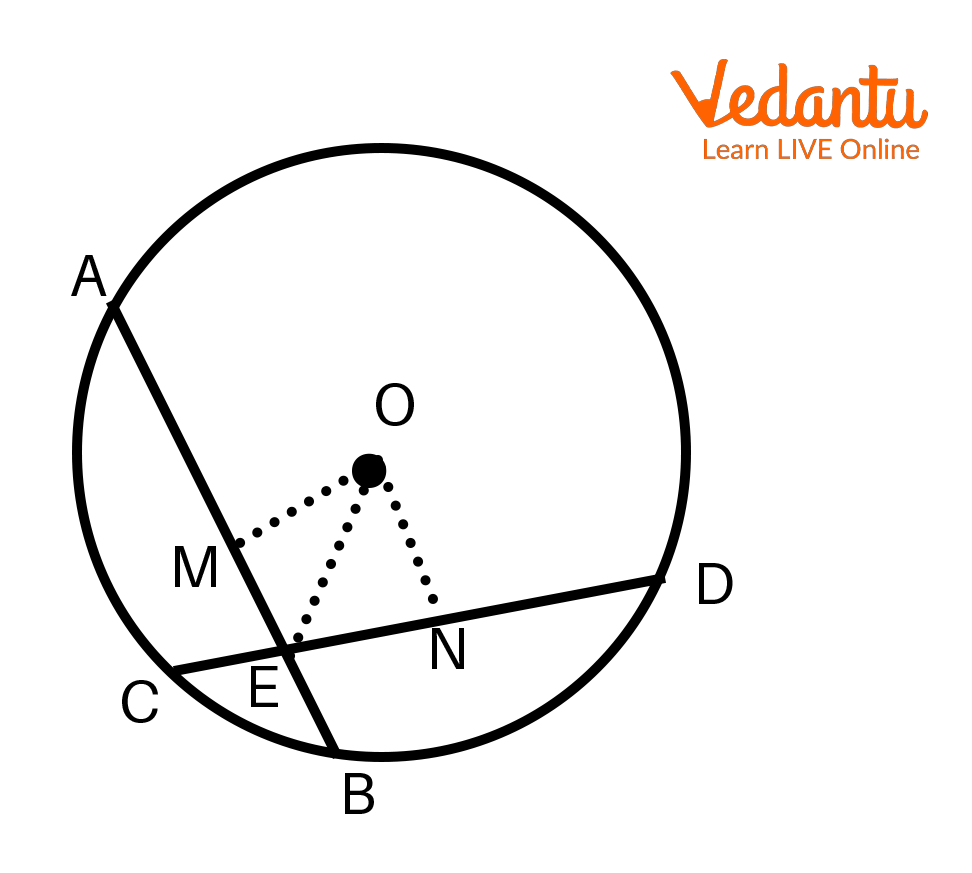The Intersection of Equal Chords

Proof:

First, join points O and E.

Then, draw a line OM from the center to the chord AB.

$\Rightarrow OM \bot AB$

$\Rightarrow$M bisects AB, which means M is the midpoint on AB.

$\Rightarrow AM = MB$ ---------------- (1)

Similarly, draw a line ON from the center to the chord CD.

$\Rightarrow ON \bot CD$

$\Rightarrow$N bisects CD, which means N is the midpoint on CD.

$\Rightarrow CN = ND$ ----------------- (2)

Now, we will see the proof:

As we have given AB = CD

$\Rightarrow \frac{1}{2}AB = \frac{1}{2}CD$

$\Rightarrow AM = ND$

$\Rightarrow MB = CN$

$\Rightarrow MB = AM = ND = CN$ ------------------- (3)

In $\Delta OME$ and $\Delta ONE$

$\Rightarrow \angle OME = \angle ONE = {90^ \circ }$ ( By construction)

$\Rightarrow OE = OE$ (Common side)

As the distance from the center is equal if the chords (AB=CD) are equal, we have

$\Rightarrow OM = ON$

$\therefore \Delta OME \cong \Delta ONE$ (RHS rule)

$\Rightarrow ME = EN$ ( CPCT rule) ----- (4)

Next, from (1) and (3), we will get,

$\Rightarrow AM = ND$

Add (4) on both sides, we will get,

$\Rightarrow AM + ME = EN + ND$

$\Rightarrow AE = DE$ ---------- (5)

And, subtract (5) from AB=CD

$\Rightarrow AB - AE = CD - DE$

$\Rightarrow BE = CE$

Hence, it is proven.

## Interesting Facts

• The radius of the circle bisects the chord at ${90^ \circ }$.

• When two radii join the two ends of a chord, they form an isosceles triangle.

• The converse of intersecting equal chords: Two intersecting chords of a circle make equal angles with the diameter that passes through their point of intersection then the chords are equal.

• When a line is drawn through the center of a circle that bisects the chord is perpendicular to the chord.

## Important Questions

1. A circular road of radius 20m. Three girls A, S and D are sitting at equal distances on its boundary, each having a toy telephone in their hands to talk to each other. Find the length of the string of each phone.

Solution:

We are given that, OS = 20m and AS = SD = DA =?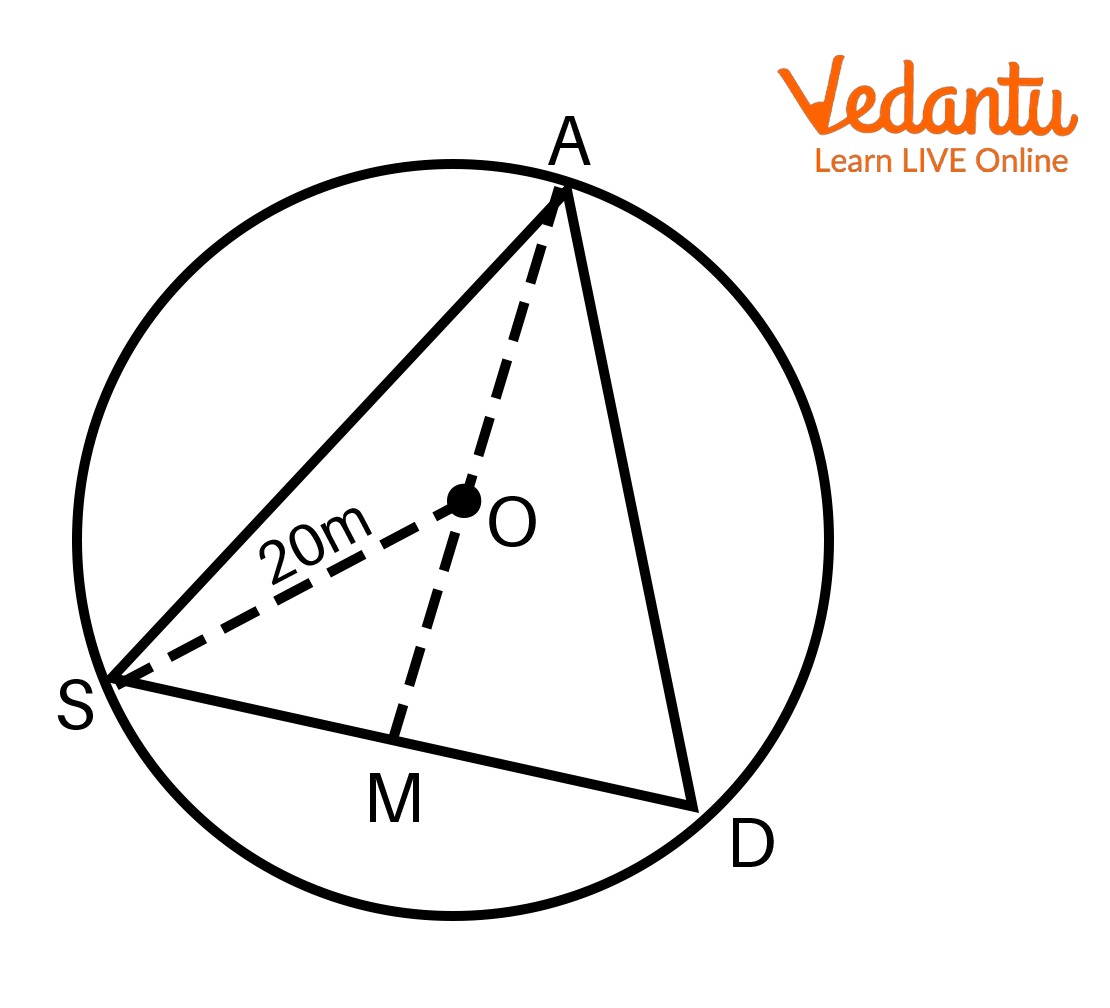From this, we can say that $\Delta ASD$ is an equilateral triangle.

$\Rightarrow OS = OA = OD = 20$

As AM is the median and altitude of $\Delta ASD$

$\Rightarrow$M is the midpoint of SD --------- (1)

And point O is the centroid.

$\therefore$O divides AM in 2:1

$\Rightarrow \frac{{OA}}{{OM}} = \frac{2}{1}$

$\Rightarrow \frac{{20}}{{OM}} = \frac{2}{1}$

$\Rightarrow OM = 10m$

Next, from this we can see that $\Delta OMS = {90^ \circ }$

$\Rightarrow O{S^2} = O{M^2} + S{M^2}$

$\Rightarrow {(20)^2} = {(10)^2} + S{M^2}$

$\Rightarrow S{M^2} = 300$

$\Rightarrow SM = 10\sqrt 3 m$

From (1) we can say that,

$\Rightarrow SM = DM = 10\sqrt 3 m$

$\Rightarrow SD = 2SM = 2 \times 10\sqrt 3$

$\Rightarrow SD = 20\sqrt 3$m

Thus, we have $AS = SD = AD = 20\sqrt 3$m.

2. In this circle with center O, the radius is 8 cm and XY=PQ=12 cm. Find the lengths of OS and OT.

Solution:

According to the given information, we will get,A Perpendicular on the Chord

Given: OY=OQ=8 cm ------- (1)

And, XY = 12 cm

According to the figure,

$\Rightarrow XS + SY = SY$

Also, the perpendicular drawn from the center to the chord bisects the chord

$\Rightarrow XS = SY$

Next, $SY + SY = XY$

$\Rightarrow SY = \frac{1}{2} \times XY$

$\Rightarrow SY = \frac{1}{2} \times 12$

$\Rightarrow SY = 6$ cm ----- (2)

In $\Delta OSY$, using Pythagoras Theorem,

$\Rightarrow O{Y^2} = S{Y^2} + O{S^2}$

$\Rightarrow O{S^2} = O{Y^2} - S{Y^2}$

$\Rightarrow O{S^2} = {8^2} - {6^2}$

$\Rightarrow O{S^2} = 28$

$\Rightarrow OS = 2\sqrt 7$cm -------- (3)

According to the question, XY=PQ

$\Rightarrow OS = OT$ ( Equal chords of a circle are equidistant from the center)

$\Rightarrow OT = 2\sqrt 7$ cm -------- (4)

Thus, the length of OS and OT is $2\sqrt 7$ cm.

3. If a line intersects two concentric circles (circles with the same center) with center O at A, B, C and D. Prove that AB = CD.

Solution:

We have taken point E which joins from the center. According to the given information, we get the following figure:Two concentric circles

As $OE \bot AD$

$\Rightarrow AE = ED$ ----- (1)

And, $OE \bot BC$

$\Rightarrow BE = EC$ ----- (2)

From (1) – (2), we will get,

$\Rightarrow AE - BE = ED - EC$

$\Rightarrow AB = CD$

Thus, it is proved.

## Conclusion

The article summarizes the theorem of equal chords and their distances from the center i.e. equal chords are equidistant from the center and then it's converse too. Then we learned the theorem of the intersection of equal chords too. Also, this article has solved examples to understand the concepts easily and see that the largest chord of the circle is known as the diameter.

## Practice Questions

1. We have two circles of radii 5 cm and 3 cm which intersect at two points and the distance between their centers is 4 cm. Find the length of the common chord.

A. 3 cm

B. 6 cm

C. 4 cm

2. Equal chords of a circle make equal angles at the center of a circle. Is this statement true?

A. Yes

B. No

C. Can’t determined

1) B

2) A

## FAQs on Equal Chords and their Distances from Center

1. Why is the diameter the longest chord of a circle?

A circle has an infinite number of chords. When the chords move closer to the center, then the length of the chords increases. This means the chord nearer to the center of a circle is the longest chord. So the chord which is away from the center is the least. Thus, the longest chord of a circle is called as diameter which passes through the center of a circle.

2. Does a circle has an infinite number of equal chords?

A circle has only a finite number of equal chords.

3. Can we say that every chord is the radius of the circle?

We know that the line which connects the point from the center of the circle is called the radius. And, any two points on the circumference join to form the chord. Thus, we can say that a radius is not the chord of the circle.